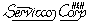Sampling Plans Site Map

Specifications & Support Information:

Validation of Software ] [ Validation of TSS Plans ] Customer Service ] Windows Compatibility ] Year 2000 ] Off-Site Information ]

 Validation of TSS visitors=This page describes the validation of TSS sampling plans for variables by simulating TSS decisions in MS Excel .

Sections:

TSS Sequential Sampling Plans:

TSS is a method of developing sequential decision rules. The TSS application to situations with unknown standard deviation is different enough from the known-sigma technique to warrant separate analyses for the two.

Sampling plans were applied to "artificial" data. MS-Excel was used to produce the data as random samples from a specified normal distribution. The following TSS sampling plan for unknown standard deviation was simulated with Excel:Lower individual specification limit (LISL) =0.00Producers risk, Alpha=0.05Consumers risk, Beta=0.05AQL=0.01 (fraction nonconforming to LISL)RQL=0.12 (fraction nonconforming to LISL).

PROCEDURE: The decisions of the TSS sampling plan were compared to the decisions of the matched fixed-n plan. Both plans had the same LISL, alpha, beta, AQL, and RQL. Both types of sampling plan received the same samples.

The accept/reject decisions were carried out with populations at the AQL and RQL points. The Excel program made three runs of 10,000 decisions  each at the AQL and three similar runs at the RQL.

Each accept/reject decision involved generating fixed-n=21 data points.

The probability of acceptance for each point (mean) on the oc curve was calculated as Pa = (number of acceptances)/(total decisions).

Table 1: The numbers in the body of the table represent measurements by simulation of the producers risk (alpha).

 Simulation with the population at AQL=0.01 The specified alpha was 0.05 Number of Decisions TSS Fixed-n Difference 10,000 0.0377 0.0384 0.0007 10,000 0.0401 0.0404 0.0003 10,000 0.0384 0.0380 0.0009

Discussion: in table 1, the TSS and Fixed-n probabilities are close to the specified producers risk of 0.05.

Table 2: The numbers in the body of the table represent measurements by simulation of the consumers risk (beta).

 Simulation with the population at RQL=0.12 The specified beta was 0.05 Number of Decisions TSS Fixed-n Difference 10,000 0.0517 0.0490 0.0027 10,000 0.0550 0.0508 0.0042 10,000 0.0567 0.0542 0.0025

Discussion: In table 2, the TSS and Fixed-n probabilities are close to the specified consumers risk of 0.05.

Results for TSS Known-Sigma plans:

The computer program SAS (Statistical Analysis System) was used by 3M Company to simulate known-sigma TSS sampling plans.  The OC-Curve and the ASN-curve were simulated at eleven points (population means) each. At each point, the SAS program made 5,000 decisions for TSS sequential, Fixed-n, and SPR sequential sampling plans.

You can contact H & H Servicco Corp. for free summary the results of this study.

The report shows that known sigma TSS sequential sampling plans are more effective than either fixed-n or SPR sequential sampling plans.